GeeksforGeeks App
Open AppBrowser
Continue

# Difference Between Array Formula and Regular Excel Formula

An array formula performs multiple calculations and processes multiple values at once, the array formula performs operations depending on the condition we have used with the array formula. Whereas the Regular excel formula performs operations on an individual value. Before understanding the difference between the two, let’s first understand the definitions of each formula in detail.

## Regular Excel Formula

In order to apply the regular excel formula, we require a dataset. For example, if we have 10 different numbers and we are asked to find out the top 3 smallest numbers.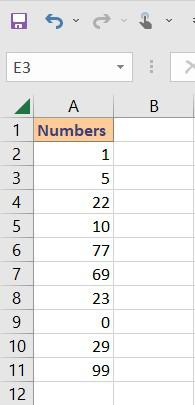If we will use the regular excel formula, =SMALL(A2:A11, 3) we will get only the 3rd smallest value. Note that, we will not get all 3 smallest values instead we will get only the 3rd smallest value from our data source.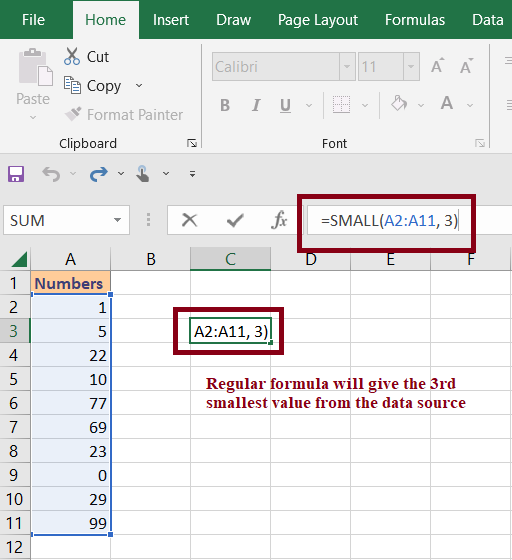## Array Formula

Using the array formula, we can easily find the top 3 smallest values from our data source in a single step. For this Select 3 Cells > Add Following Array Formula =SMALL(A2:A11,{1;2;3}) > Ctrl + Shift + Enter. As we click Ctrl + Shift + Enter, Excel will convert our formula into an array formula. And, find the top 3 smallest values from our data source.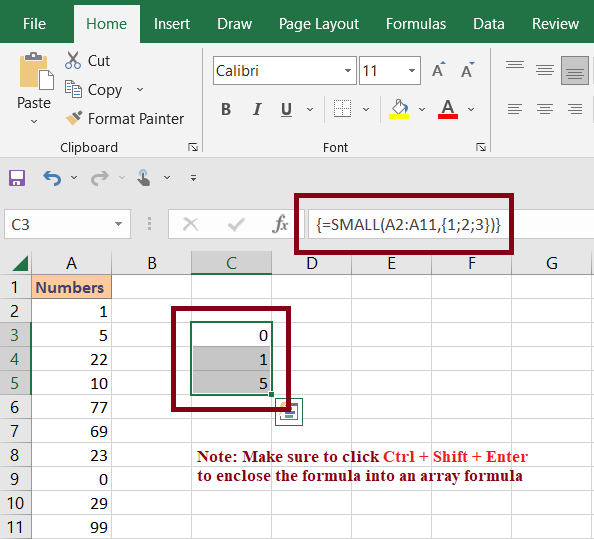### What is Array Formula?

An Array Formula in excel is a very powerful tool that allows us to perform multiple calculations at once. Along with this it can also be used to do single or multiple calculations with a fixed range multiple times.

Note: The main difference between formula and array formula is that, an array formula perform multiple calculation over an array of cells or column at once.

Before moving further let us understand what is arrays in excel. In general, the array is a collection of items. These items can be plain texts or numbers which are present in single rows or columns. It can also exist in multiple rows and columns which makes it a 2-dimensional array.

### How to Insert Array in Excel

In order to insert an array in excel, we need to select the number of columns equivalent to the number of items we want to insert. For example, if we want to insert 3 items we need to select 3 rows after that we need to insert the data in the formula bar followed by the equal (=) symbol, and then we need to press CTRL + SHIFT+ ENTER, this command will create an array in excel. In excel, we represent array as {“geeks”, “for”, “geeks”}.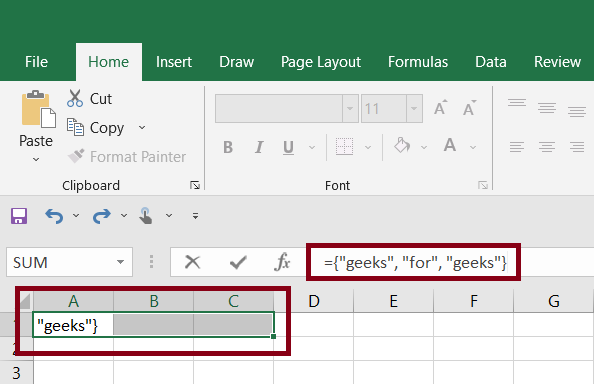Fig4- Insert array in excel

Once we click CTRL + SHIFT + ENTER, excel we automatically enter the 3 different items in 3 different rows.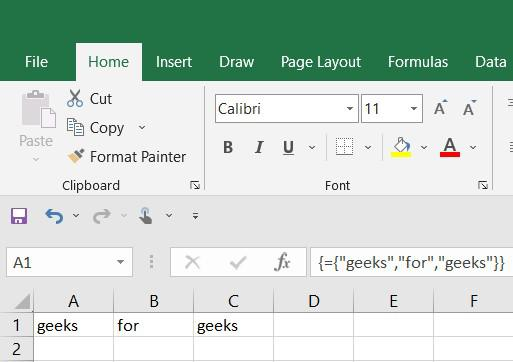Example:

In this example, we will use the following random sales for different products and we will calculate the sub-total and total for all the different products using the array formula. For this, we are going to use the following dataset.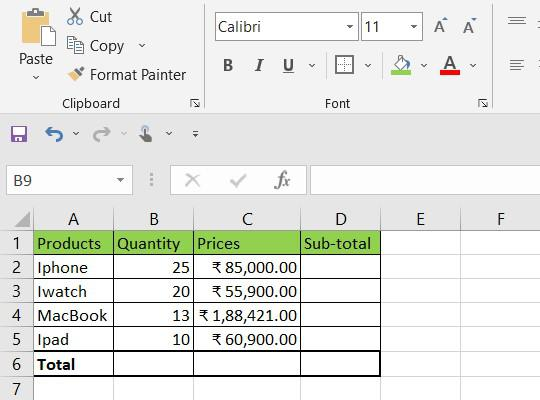### Calculating Sub-Total and Total

In order to calculate the sub-total and total we can simply calculate sub-total(B*C) and then calculate the total by adding each row. But if our dataset is having thousands of entries it became very difficult to use this method. We can reduce this operation by using the array formula as excel stores immediate results in memory rather than in additional columns.

### Steps to use Array Formula

Step 1: Calculate Subtotal

In order to calculate the sub-total, first we will select the empty cells for all the rows(Here, it is D2:D5) and then we will use the following array formula.

=(B2:B5*C2:C5)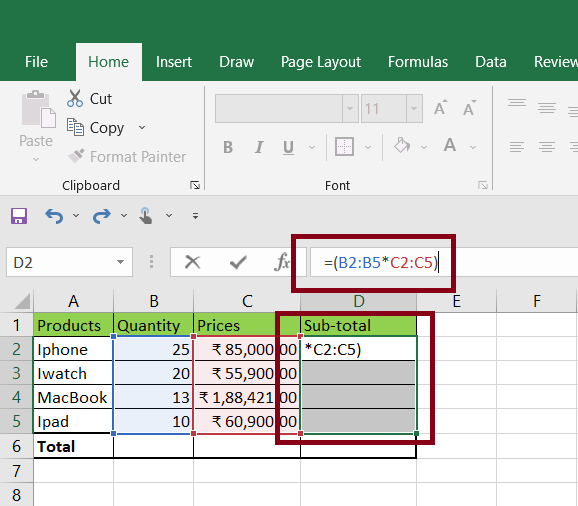After this we need to press CTRL + SHIFT + ENTER, this will enclose our condition in the array formula and we will get the sub-total for each product.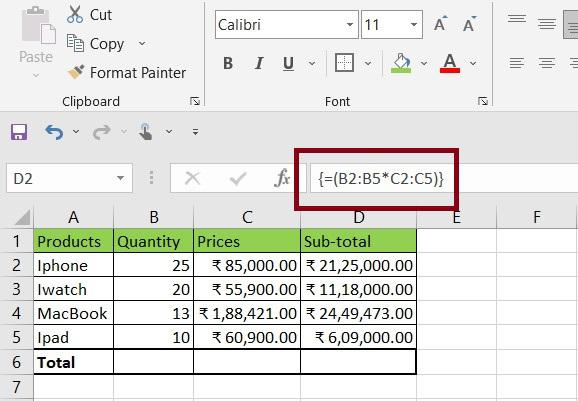Fig8 – Output

Step 2: Calculate the Total

Similarly, we order to calculate the total sum, first, we will select the empty cell(Here, D6) and then use the following array formula.

=SUM(B2:B5*C2:C5)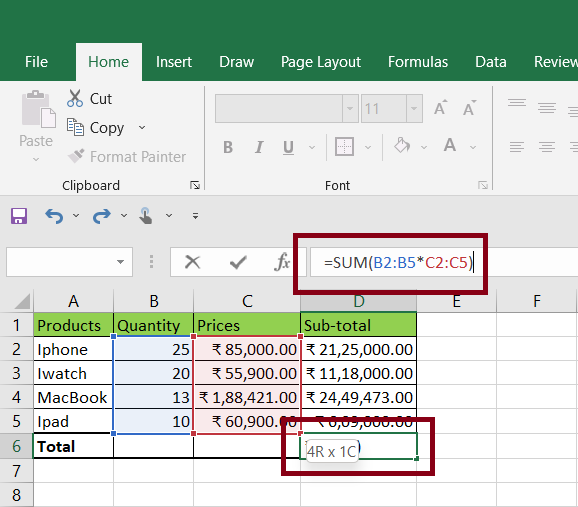After we insert our formula we need to press CTRL + SHIFT + ENTER to insert the array formula. This will in-close our condition in curly brackets and we will get the output as the total sum.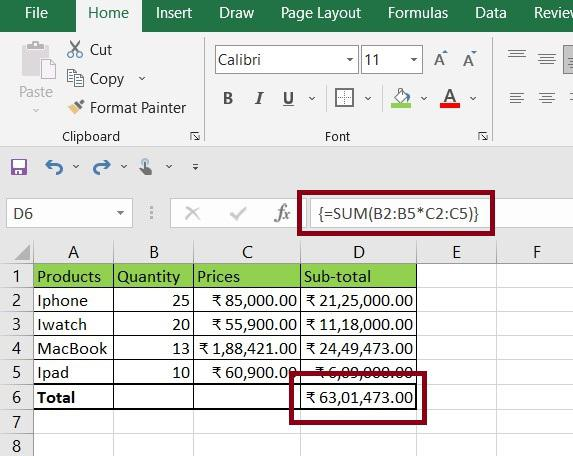## Difference between Regular Formula & Array Formula

My Personal Notes arrow_drop_up